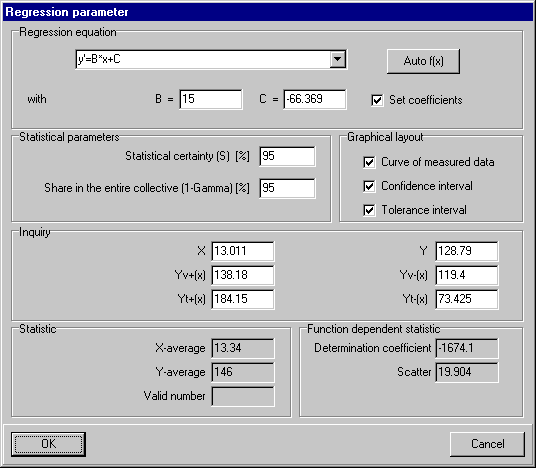# P&K 3950

## Function and Significance test

Product has been archived / Produkt wurde archiviertWith selection of the function f(x) or the button "Auto f(x)" a significance checking between the polynom formula of the first (linear regression) and the second order (quadratic regression) is performed and the quadratic formula is selected, if it is significantly (with a confidence of 95 %) better than the linear formula.

Settings and data fields

• The determination coefficient for linear and quadratic regression according to HARTUNG, page 579 and 593
• Distribution according to VDI 3950, part 1 equations 5 and 27
• Regression equation
• X- and Y-average values
• Number of measuring points
• A, B and C, the coefficients in the regression equation
• Statistical certainty
• Share of the population (1-Gamma)

Inquiry block

• x value
• y value at x
• y + confidence interval at x
• y - confidence interval at x
• y + tolerance interval at x
• y - tolerance interval at x